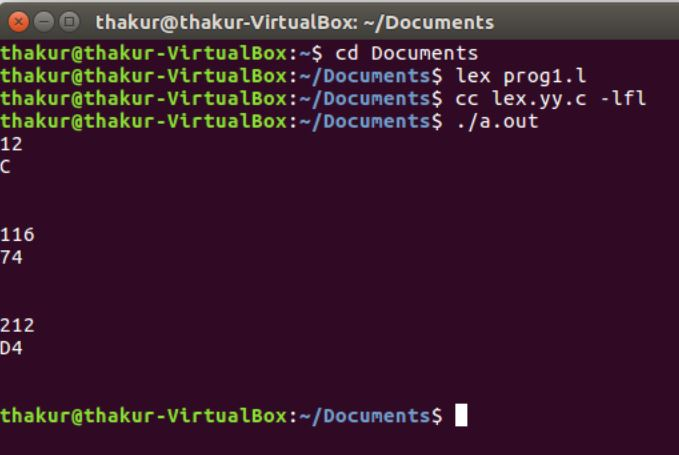# Lex program for Decimal to Hexadecimal Conversion

• Last Updated : 30 Apr, 2019

Problem: Write a Lex program for Decimal to Hexadecimal conversion.

Explanation:
Lex reads an input stream specifying the lexical analyzer and outputs source code implementing the lexer in the C programming language. The function yylex() is the main flex function which runs the Rule Section.

We provide nothing but the best curated videos and practice problems for our students. Check out the C Foundation Course and master the C language from basic to advanced level. Wait no more, start learning today!

Prerequisite: Flex (Fast lexical Analyzer Generator)

Examples:

```Input: 12
Output: C

Input: 116
Output: 74

Input: 55
Output: 37

Input: 212
Output: D4 ```

Implementation:

 `/* Lex program for decimal to hexadecimal conversion */`` ` `%{``    ``/* Definition section */``    ``#include``    ``int` `num, r, digit=0, count, pcount=0, i;``    ``char` `a;``%}`` ` `DIGIT [0-9]``/* Rule Section */``%%`` ` `{DIGIT}+ { num=``atoi``(yytext);`` ` `        ``while``(num!=0)``        ``{`` ` `            ``r=num%16;``            ``digit=``'0'``+r;``            ``if``(digit>``'9'``)``            ``digit+=7;``            ``a[count++]=digit;``            ``num=num/16;`` ` `        ``}`` ` `        ``for``(i=count-1;i>=pcount;--i)``                ``printf``(``"%c"``, a[i]);``                ``pcount=count;``        ``}`` ` `.|\n    ECHO;``        ` `%%`` ` `// driver code``int` `main()``{``    ``yylex();``    ``return` `0;``}      `

Output:My Personal Notes arrow_drop_up